6 Breaking the Coulomb barrier by electrostatic acceleration of ions in a device with a variable gridJust some idea's... (not working)

(6e idea)

With a Van der Graaff Generator a high voltage can be achieved.
In

Fig. 1. Schematic representation of a Fusor with one movable inner grid.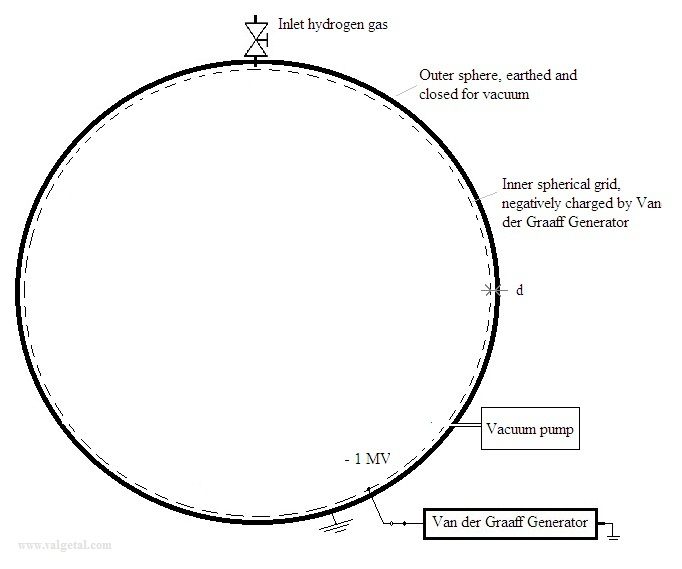The outer sphere is earthed and the inner sphere is connected to a Van der Graaff Generator (Vgg) and will be charged with a negative charge.

The configuration of the outer sphere and the inner grid could approximately be represented as a capacitor:

Fig. 2. Schematic representation of  the Fusor as capacitor.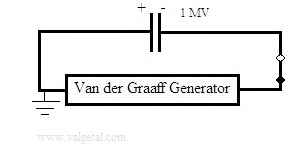Let's assume we achieve a voltage of 1 MV.  After that we disconnect the Vvg so the negative charge is trapped in the inner grid. Then suppose we could move, in one way or the other, this negative grid to the centre and making (for example)  the distance d 100x bigger (from d to 100d):

Fig. 3. Schematic representation of a Fusor with the inner grid moved towards the centre.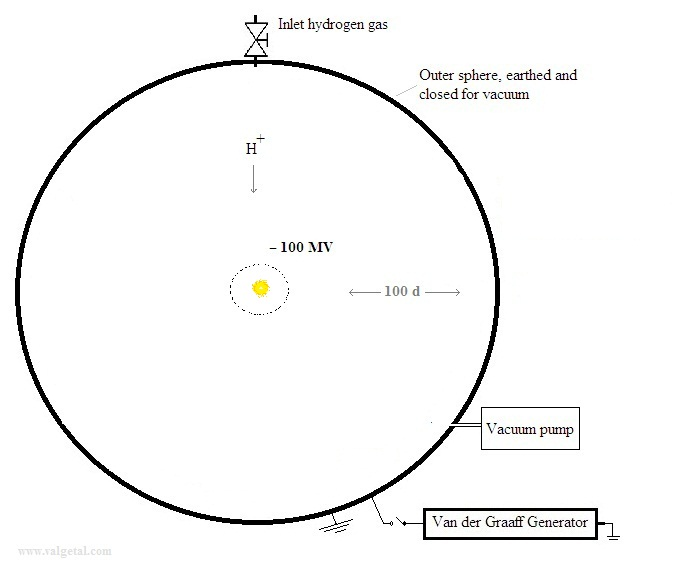If the outer sphere and the inner grid behave as an ideal capacitor, then the voltage between them would increase to 100 MV !

Fig. 4. Schematic representation of  the Fusor as capacitor;
negative charge trapped and one plate moved.

.Could we now generate any fusion reaction inside the fusor?

The boron-hydrogen fusion reaction is one of the nicest ones:

115B + 11H  -> 3 42He  + 8,68 MeV   (see en.wikipedia.org/wiki/Aneutronic_fusion)

Because no noxious neutrons are produced and the 3 positive  42He+ particles will move with high speed out of the centre increasing the voltage between the grids. They could perhaps even be used to generate directly electricity.

The strong nuclear force works until ± 2 fm = 2.10 -15 (see: wiki/Nuclear_force )

Z B  = 5  (amount of positive protons in a boron ion)

Z 1    = 1  (only one positive proton in a hydrogen ion)
k is the Coulomb's constant = 9,0×109 N m² C−2

1 eV = 1,6 . 10 -19  J
= >
Ucoul =  k . Z B . Z 1 . e 2  / r  =  9.0 . 10 9 . 5 . 1 . (1,6.10 -19 ) 2  / (2.10 -15)  = 0,6.10 -12  Nm =    0,6 .10 -12   J  =  3,6.10 6  eV

(see: wiki/Coulomb_barrier )

So to break the coulomb barrier between a boron nucleus and hydrogen nucleus about 4.10 6  eV energy is needed (note: according this very simple calculation).  If a hydrogen ion is accelerated from about the outer sphere to the centre and achieves a kinetic energy of 100 MeV, this would be more than enough to cause a nuclear fusion reaction, if it would collide with a right angle with a boron nucleus.

Questions:

Can the inner grid be considered as a moving plate of a capacitor?

How do we move and shrink the grid to the centre and how will it stay there?

Maybe use a Hoberman sphere ?

Perhaps we could use inside the fusor a mix of hydrogen and boron ions?

Or we make the inner grid of a boron compound and let the hydrogen ions collide with it?

Inside the fusor in the centre we can place a kind of small ball made of a boron compound and connect it with a very good electrical insulator to the outer sphere?

Perhaps inside the fusor we place some kind of balloon with a little bit hydrogen gas. When we pump the fusor empty it will increase in size. On the surface of the balloon there is a layer of boron compound: the inner grid. Then we pinch the balloon and it will shrink.... I don't think it will work..

Let us consider the sphere and grid not as a capacitor, but just as charged spheres:

We apply GaussThe voltage will even more increase....

Does it take energy (force) to move the inner grid to the centre?

There will be formed a positive charge on the inside of the outer sphere when we charge the inner grid negatively.
Let's apply Gauss over a spherical surface just inside the outer sphere: Any electric field generated by the positive charge on the inside of the outer sphere should be radial and pointing towards (or from) the centre (because of symmetry). But the surface integral over this spherical surface should be zero, because there is no positive charge inside it. So the positive charge on the inside of the outer sphere will not generate an electric field inside the outer sphere, and the negative grid will not feel an electric force exerted by the positive charge .

Fig. 4. Schematic representation of  the outer sphere and the inner grid, with two surface integrals.

ºº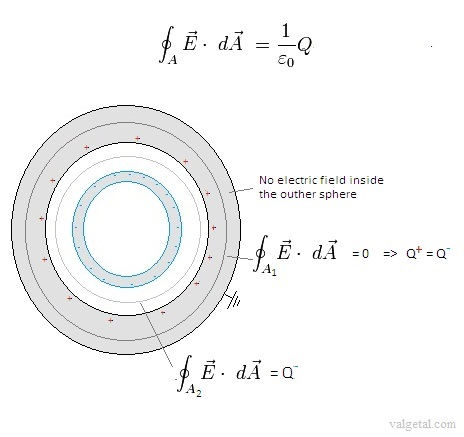But maybe the negative charge on the inner grid will exert forces on itself, and therefore energy (force) will be needed to move the inner grid to the centre?

Let's divide the negative charge Q in 1000 small charges. Move 1/1000 Q from r-d to r-100d. The rest of the charges can mathematically be considered to be in the centre. The labour that is needed to move this charge (1/1000 Q) is:

(r=1,00 m  ,  d = 0,005 m , Q = 0,022 Coulomb )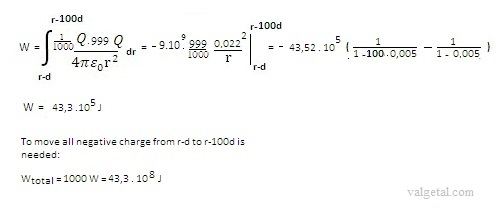Wtotal = 43.108 / 1000.3600 = 1194 kwh x 14 cent = 167 € = 123 ltr petrol

The assumption that the rest of the charges can mathematically be considered to be in the centre is not correct (I suppose). When the first small charge is moved towards the centre of the negatively charged inner grid, it will not feel any force upon it because inside the grid there isn't any electric field. But when we have moved already almost all charge towards the centre, then the last charge yes will feel a repulsive force when it is moved towards the centre.

I still not know if it takes energy to move the negative charged grid towards de centre or not, but technically I do not see a way to do this and neither how the grid can stay in the centre....

Remark: If an ion passes a voltage difference of more then 100.10V it looses a lot of energy, more then the energy generated by a possible fusion... so this device will never generate a net energy..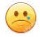(Jan. 2013) (Jan. 2013) (Jan. 2013) (3 Febr. 2013) (6 Febr. 2013) (8 Febr. 2013) (16 Jan. 2014) (17 Jan. 2014) (20 Jan. 2014) ( Febr. 2014- 2016) Computer simulation tenth idea (2014-2016) Eleventh idea   (2016.. 2017)8 February 2013      by  Rinze Joustra        www.valgetal.com